Courses

# JEE Main Previous year questions (2016-20): Surface Chemistry Notes | EduRev

## JEE : JEE Main Previous year questions (2016-20): Surface Chemistry Notes | EduRev

The document JEE Main Previous year questions (2016-20): Surface Chemistry Notes | EduRev is a part of the JEE Course Chemistry for JEE.
All you need of JEE at this link: JEE

Q.1. The flocculation value of HCl for arsenic sulphide sol. is 30 m mol L−1. If H2SO4 is used for the flocculation of arsenic sulphide, the amount (in mgs), of H2SO4 in 250 mL required for the above purpose is ____________.    (2020)
(Molecular mass of H2SO4 = 98 g mol–1)

Ans. (37)
Given, the flocculation value of HCl for arsenic sol is 30 m mol L–1.
⇒ 1 L of arsenic sol required 30 m mol of HCl.
We know,
n(H2SO4) = 2 x nf  (HCI)
⇒1 L of arsenic sol required 15 m mol of H2SO4.
Thus, for 250 mL of arsenic sol, the amount of H2SO4 required is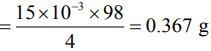= 37mg.

Q.2. As per Hardy-Schulze formulation, the flocculation values of the following for ferric hydroxide sol are in the order    (2020)
(1) K3 [Fe(CN6)] < K2CrO4 < KBr = KNO3 = AlCl3
(2) K3 [Fe(CN6)] < K2CrO4 < AlCl3 < KBr = KNO3
(3) AlCl3 > K3 [Fe(CN6)] > K2CrO4 > KBr = KNO3
(4) K3[Fe(CN6)] > AlCl3 > K2CrO4 > KBr > KNO3
Ans.
(3)
We know,
Coagulation value or Flocculation value ∝ 1/Coagulation power
AlCl3 > K3[Fe(CN)6] > K2CrO4 > KBr = KNO3

Q.3. A mixture of gases O2, H2 and CO are taken in a closed vessel containing charcoal. The graph that represents the correct behavior of pressure with time is    (2020)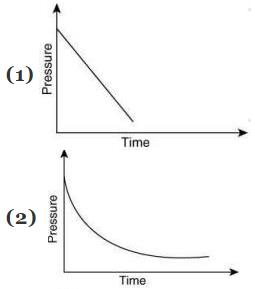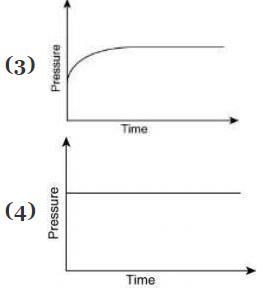Ans. (2)
As the gases get adsorbed on the surface of charcoal, with time, the number of moles of free gases in the container decreases, thus, the pressure of gas with decrease exponentially. Hence, the correct representation of behavior of pressure with time is: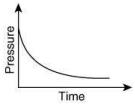Q.4. Adsorption of a gas follows Freundlich adsorption isotherm. In the given plot, x is the mass of the gas adsorbed on mass m of the adsorbent at pressure p. x/m is proportional to:    (2019)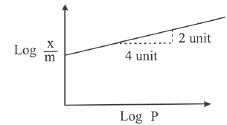(1) p2
(2) p1/4
(3) p1/2
(4) p
Ans.
(3)
In Freundlich adsorption isotherm the extent of adsorption (x/m) of a gas on the surface of a solid is related to the pressure of the gas (P) which can be formulated as: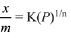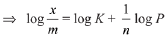In the given plot, the slope between log x/m versus log P = 2/4 = 1/2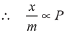Q.5. For coagulation of arsenious sulphide sol, which one of the following salt solution will be most effective?    (2019)
(1) BaCl2
(2) AICI3
(3) NacI
(4) Na3PO4
Ans.
(2)
For coagulation of a negatively charged arsenious sulphide sol, the cation which is in higher oxidation state will be most effective.

Q.6. Which of the following is not an example of heterogeneous catalytic reaction?    (2019)
(1) Ostwald’s process
(2) Combustion of coal
(3) Hydrogenatoin of vegetable oils
(4) Haber’s process
Ans.
(2)
C + O2 → CO2
No catalyst is required.
ln Ostwald process, Haber’s process and hydrogenation of vegetable oils, catalyst (solid) such as Pt/Rh, Fe and Ni are used.

Q.7. Haemoglobin and gold sol are examples of:    (2019)
(1) positively and negatively charged sols, respectively
(2) positively charged sols
(3) negatively charged sols
(4) negatively and positively charged sols, respectively
Ans.
(1)
Haemoglobin and gold sol (metal) are examples of positive and negative sols respectively.

Q.8. An example of solid sol is:    (2019)
(1) Paint
(2) Gem stones
(3) Butter
(4) Hair cream
Ans.
(2)
Gem stones are solid sol.

Q.9. Among the colloids cheese (C), milk (M) and smoke (S), the correct combination of the dispersed phase and dispersion medium, respectively is:    (2019)
(1) C : liquid in solid; M : liquid in solid; S : solid in gas
(2) C : liquid in solid; M : liquid in liquid; S : solid in gas
(3) C : solid in liquid; M : liquid in liquid; S : gas in solid
(4) C : solid in liquid; M : solid in liquid; S : solid in gas
Ans.
(2)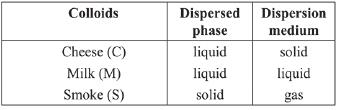Q.10. Given gas critical temperature/K for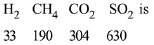On the basis of data given above, predict which of the following gases shows least adsorption on a definite amount of charcoal?    (2019)
(1) SO2
(2) CH4
(3) CO2
(4) H2
Ans.
(4)
Smaller the value of critical temperature of gas, lesser is the extent of adsorption.
∴ Least adsorption is shown by H2 (least critical temperature).

Q.11. Among the following, the false statement is:    (2019)
(1) It is possible to cause artificial rain by throwing electrified sand carrying charge opposite to the one on clouds from an aeroplane.
(2) Tyndall effect can be used to distinguish between a colloidal solution and a true solution.
(3) Lyophilic sol can be coagulated by adding an electrolyte.
(4) Latex is a colloidal solution of rubber particles which are positively charged.
Ans.
(4)
Latex is a colloidal solution of negatively charged rubber particles.

Q.12. Adsorption of a gas follows Freundlich adsorption isotherm, x is the mass of the gas adsorbed on mass m of the adsorbent. The plot of x/m versus log p is shown in the given graph. x/m is proportional to:    (2019)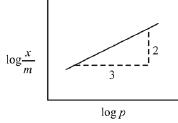(1) p2/3
(2) p3/2
(3) p3
(4) p2
Ans.
(1)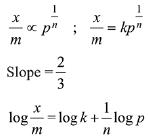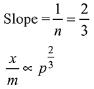Q.13. 0.27 g of a long chain fatty acid was dissolved in 100 cm3 of hexane. 10 mL of this solution was added dropwise to the surface of water in a round watch glass. Hexane evaporates and a monolayer is formed. The distance from edge to centre of the watch glass is 10 cm. What is the height of the monolayer?
[Density of fatty acid = 0.9 gcm-3 ; π = 3]    (2019)
(1) 10-6 m
(2) 10-8 m
(3) 10-2 m
(4) 10-4 m
Ans.
(1)
Given:
0.27 g is present in 100 cm3 of hexane
∴ 10 mL of aqueous solution contains only 0.027 g acid.
Volume of 0.027 g acid = 0.027/0.9 mL
∴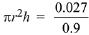(given r = 10 cm, π = 3)
∴ h = 10-4 cm = 10-6 m

Q.14. Match the catalysts (Column I) with products (Column II).    (2019)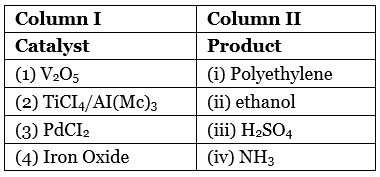(1) (i)-(iii); (B)-(iv); (C)-(i); (D)-(ii)
(2) (i)-(ii); (B)-(iii); (C)-(i); (D)-(iv)
(3) (i)-(iii); (B)-(i); (C)-(ii); (D)-(iv)
(4) (i)-(iv); (B)-(iii); (C)-(ii); (D)-(i)
Ans.
(3)
(A) V2O: Preparation of H2SO4 in contact process
(B) TiCl+ Al(Me): Polyethylene (Ziegler-Natta catalyst)
(C) PdCl2 : Ethanal (Wacker’s process)
(D) Iron oxide : NHin (Haber’s process)

Q.15. The aerosol is a kind of colloid in which:    (2019)
(1) solid is dispersed in gas
(2) gas is dispersed in solid
(3) gas is dispersed in liquid
(4) liquid is dispersed in water
Ans.
(1)
In aerosol, the dispersion medium is gas while the dispersed phase can be both solid or liquid.

Q.16. A gas undergoes physical adsorption on a surface and follows the given Freundlich adsorption isotherm equation x/m = kp0.5 Adsorption of the gas increases with:    (2019)
(1) Decrease in p and increase in T
(2) Decrease in p and decrease in T
(3) Increase in p and decrease in T
(4) Increase in p and increase in T
Ans.
(3)
Freundlich adsorption is applicable for physical adsorption. The variation of extent of adsorption with (i) Pressure and (ii) Temperature is given by the following curves.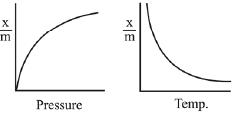Hence, extent of adsorption increases with increase in pressure and decrease in temperature.

Q.17. The correct option among the following is:    (2019)
(1) Colloidal medicines are more effective because they have small surface area.
(2) Addition of alum to water makes it unfit for drinking
(3) Colloidal particles in lyophobic sols can be precipitated by electrophoresis.
(4) Brownian motion in colloidal solution is faster if the viscosity of the solution is very high.
Ans.
(3)
The process of electrophoresis is used to precipitate colloidal particles in lyophobic sols.

Q.18. Peptization is a:    (2019)
(1) process of bringing colloidal molecule into solution
(2) process of converting precipitate into colloidal solution
(3) process of converting a colloidal solution into precipitate
(4) process of converting soluble particles to form colloidal solution

Ans. (2)
Peptisation is the process of converting a precipitate into a colloidal sol by shaking it with dispersion medium in the presence of small amount of electrolyte. It is also known as deflocculation.

Q.19. Among the following, the INCORRECT statement about colloids is:    (2019)
(1) They can scatter light.
(2) They are larger than small molecules and have high molar mass.
(3) The osmotic pressure of a colloidal solution is of higher order than the true solution at the same concentration.
(4) The range of diameters of colloidal particles is between 1 and 1000 nm.
Ans.
(3)
Due to the association of solute molecule till they acquire colloidal dimensions, the osmotic pressure of a colloidal solution is of lower order than that of true solution at the same concentation.
π = iCRT
i (vant hoff factor) is less in colloidal solution than true solution. So, π (osmotic pressures) will also be less in colloidal solution.

Q.20. Which one of the following is not a property of physical adsorption?    (2018)
(1) Higher the pressure, more the adsorption
(2) Greater the surface area, more the adsorption
(3) Lower the temperature, more the adsorption
Ans.
(4)

Q.21. Among the following, correct statement is:    (2018)
(1) Sols of metal sulphides are lyophilic.
(2) Brownian movement is more pronounced for smaller particles than for bigger-particles
(3) One would expect charcoal to adsorb chlorine more than hydrogen sulphide.
(4) Hardy Schulze law states that bigger the size of the ion is, the greater is its coagulating power.
Ans. (3)

Q.22. Adsorption of a gas on a surface follows Freundlich adsorption isotherm. Plot of log x/m versus log p gives a straight line with slope equal to 0.5, then : (x/m is the mass of the gas adsorbed per gram of adsorbent)    (2017)
(1) Adsorption is proportional to the square root of pressure.
(2) Adsorption is proportional to the square of pressure.
(3) Adsorption is proportional to the pressure.
(4) Adsorption is independent of pressure.

Ans. (1)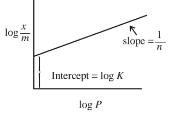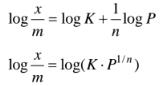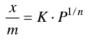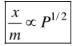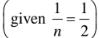Q.23. A particular adsorption process has the following characteristics:
(i) It arises due to vander Waals forces and
(ii) it is reversible.
Identify the correct statement that describes the above adsorption process:    (2016)
(1) Enthalpy of adsorption is greater than 100 kJ mol-1.
(3) Adsorption increases with increase in temperature.
(4) Energy of activation is low.
Ans.
(4)
Adsorption aries due to Vander waal forces & is reversible, hence it should be physisorption (physical adsorption).
(i) Enthalpy of physisorption is low (20 – 40 kJ/mol)
(ii) In physisorption multimolecular layer form.
(iii)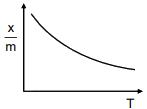Physisorption decreases with increase in temperature.
(iv) Physisorption required number activation energy.

Q.24. Match the items in Column I with its main use listed in Column II:    (2016)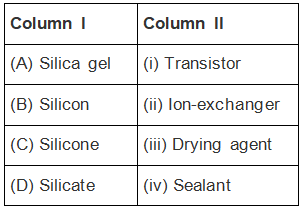(1) (A)-(iii), (B)-(i), (C)-(iv), (D)-(ii)
(2) (A)-(ii), (B)-(i), (C)-(iv), (D)-(iii)
(3) (A)-(iv), (B)-(i), (C)-(ii), (D)-(iii)
(4) (A)-(ii), (B)-(iv), (C)-(i), (D)-(iii)
Ans.
(1)
Based on theoretical fact.

Q.25. Gold numbers of some colloids are : Gelatin : 0.005 – 0.01, Gum Arabic : 0.15 – 0.25 ; Oleate : 0.04 – 1.0 ; Starch : 15 – 25. Which among these is a better protective colloid?    (2016)
(1) Gelatin
(2) Starch
(3) Gum Arabic
(4) Oleate
Ans.
(1)
Lower the gold number, more will be protective power of colloid.

Offer running on EduRev: Apply code STAYHOME200 to get INR 200 off on our premium plan EduRev Infinity!

## Chemistry for JEE

198 videos|450 docs|370 tests

,

,

,

,

,

,

,

,

,

,

,

,

,

,

,

,

,

,

,

,

,

;